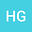•In this paper, we show a regularity criteria for three dimensional nematic liquid crystal flows. More precisely, we prove that the strong solution $(u,d)$ can be extended beyond $T$, provided $\nabla u_{3}\in{L^{p}(0,T; L^{q}(\mathbb{R}^{3}))}$, $\frac{2}{p}+\frac{3}{q}\leq{\frac{19}{12}+\frac{1}{2q}}(\frac{30}{19}3)$ with some conditions about the orientation field $\nabla_{h}d\in{L^{\alpha}(0,T; L^{\beta}(\mathbb{R}^{3}))}$, $\frac{2}{\alpha}+\frac{3}{\beta}\leq{\frac{3}{4}+\frac{1}{2\beta}}(\beta>{\frac{10}{3}})$.